# Python

OS、QNX、VMS、Psion、Acom RISC OS、VxWorks、PlayStation、Sharp Zaurus、Windows

# 应用

Web编程：应用的开发语言，支持最新的XML技术。

pymo引擎：PYMO全称为python memories off，是一款运行于Symbian S60V3,Symbian3,S60V5, Symbian3,
Android系统上的AVG游戏引擎。因其基于python2.0平台开发，并且适用于创建秋之回忆（memories
off）风格的AVG游戏，故命名为PYMO。

# 官方代码样式标识符 由字母，数值和下划线组成且`不能以数字`开头，区分大小写。

`import keyword`
`keyword.kwlist`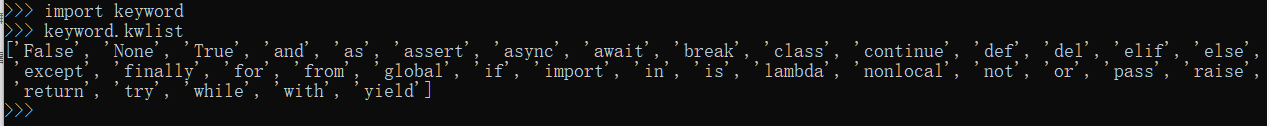False ）内置常量
None ）内置常量
True ）内置常量
and）用于表达式运算，逻辑与操作
as）用于类型转换
assert）断言，用于判断变量或条件表达式的值是否为真
async）3.7.0新增
await）3.7.0新增，只能在通过async修饰的函数中，否则报错SyntaxError
break）中断循环语句的执行
class）用于定义类
continue）继续执行下一次循环
def）用于定义函数或方法
del）删除变量或序列的值
elif）条件语句，与if，else结合使用
else）条件语句，与if，elif结合使用
except）包含捕获异常后的操作代码块，与try，finally结合使用
for）for循环语句
finally）3.7.0新增，用于异常语句，出现异常后，始终要执行finally包含的代码块，与try，except结合使用
from）用于导入模块，与import结合使用
global）定义全局变量
if）条件语句，与elif，else结合使用
import）用于导入模块，与from结合使用
in）判断变量是否在序列中
is）判断变量是否为某个类的实例
lambda）定义匿名函数
nonlocal）用于声明，修改嵌套作用域（enclosing作用域，外层非全局作用域）中的变量
not）用于表达式运算，逻辑非操作
or）用于表达式运算，逻辑非操作
pass）空的类，方法或函数的占位符
raise）异常操作输出
return）用于从函数返回计算结果
try）包含可能会出现异常的语句，与except，finally结合使用
while）循环语句
with）简化python的语句
yield）用于从函数依次返回值

1,在python中，定义变量时是不需要指定变量的类型的

`str` 表示是一个字符串类型

``````name = "小明"
``````

`int` 表示是一个整数类型

``````age = 18
``````

`bool`表示是布尔类型，真`True` 或假`False`

``````gender = True
``````

`float` 表示是一个小数类型（浮点数）

``````height = 1.75
weight = 75.0
``````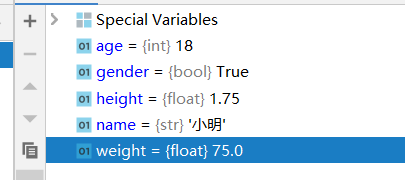2,两个字符串变量之间不能直接使用乘法

``````price_str = input("苹果的单价：")
weight_str = input("苹果的重量：")
# 1> 将价格转换为小数
price = float(price_str)
# 2> 将重量转换成小数
weight = float(weight_str)
money = price * weight
print(money)
``````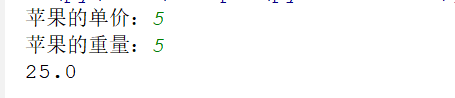``````price = float(input("苹果的单价："))
weight = float(input("苹果的重量："))
money = price * weight
print(money)
``````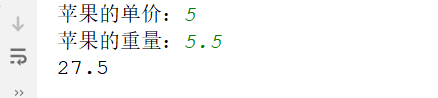3,在字符串内部，%s表示字符串替换，%d表示用整数替换，有几个%占位符，后面就跟几个变量或值，顺序要对应好。如果只有一个%，那么括号可以省略。（十进制 %06d，表示显示位数用0补全， %02f 表示小数点后只显示两位）

``````name = "小明"
print("我的名字叫 %s，请多多关照！" % name)
student_no = 100
print("我的学号是 %04d" % student_no)
price = 8.5
weight = 7.6
money = price * weight
print("输出苹果单价 %.02f 元/斤, 购买了 %.02f 斤, 需要支付 %.02f 元" %(price,weight,money))
scale = 0.25
print("数据比例是 %.2f%%" % (scale * 100))
``````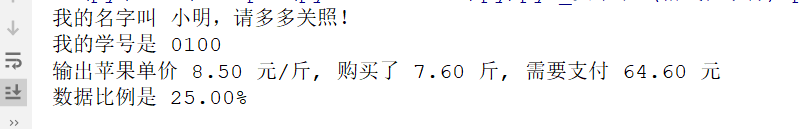4,`if`判断(条件)语句

``````age = int(input("请输入年龄:"))
if age >= 18:

print("你已成年，欢迎进入")

else:
print("禁止进入")
# 这句代码无论条件是否成立都会执行
print("这句代码什么时候执行")
``````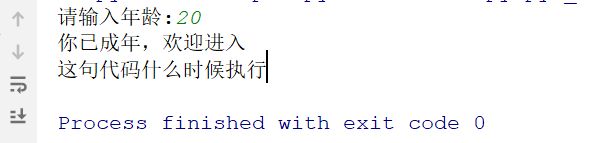5,`and,or,not`逻辑运算

``````# 1 定义一个整数变量age，判断年龄是否正确
age = 130
# 2 要求人的年龄在0 - 120 之间
if age >= 0 and age <= 120:
print("年龄正确")
else:
print("年龄不正确")
``````

``````# 定义两个整数变量 a_score,b_score,判断成绩
a_score = 40
b_score = 50
# 要求只要有一门成绩 >60 分就合格
if a_score >60 or b_score >60:
print("通过")
else:
print("继续努力")
``````

``````# 定义一个布尔型变量 is_employee, 判断是否属于本公司员工
is_employee = False
# 如果不是提示禁止入内
# 在开发中，通常希望某个条件不满足时，可以使用 not
# 如果需要拼接复杂的逻辑计算条件，同样也可能使用到 not
if not is_employee:
print("禁止入内")
``````

6,`elif`

``````# 定义holiday_name 字符串变量记录节日名称
holiday_name = "情人节"
if holiday_name == "情人节":
print("买玫瑰")
print("看电影")
elif holiday_name == "平安夜":
print("买苹果")
print("吃大餐")
elif holiday_name =="生日":
print("买蛋糕")
else:
print("每天都是节日啊")
``````

7,`if`的嵌套

``````# 定义布尔型变量 has_ticket 表示是否有车票
has_ticket = True
# 定义整数变量 knife_length 表示刀的长度，厘米
knife_length = 3
# 首先检查是否有车票
if has_ticket:
print("请安检")
# 安检，检查刀具长度
if knife_length > 20:
print("刀具过长，有 %d 厘米" % knife_length)
print("禁止入内")
else:
print("通过")
# 没有车票
else:
print("请先买票")
``````

8,石头剪刀布

``````# 导入随机工具包
# 注意：在导入工具包的时候，应该将导入的语句，放在文件的顶部
import random

# 从控制台输出要出的拳 ——石头(1)/剪刀(2)/布(3)
player = int(input("请输入你要出的拳：石头(1)/剪刀(2)/布(3)："))

# 电脑 随机 出拳
computer = random.randint(1, 3)
print("玩家选择的拳是 %d — 电脑出的拳是 %d" % (player, computer))

# 比较胜负
if ((player == 1 and computer == 2)
or (player == 2 and computer == 3)
or (player == 3 and computer == 1)):
print("胜利")
elif player == computer:
print("平局")
else:
print("失败")

``````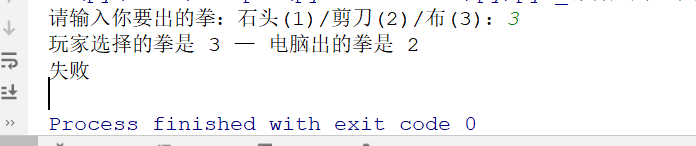9,`while`循环语句

``````# 打印 Hello Python
# 定义一个整数变量
i = 0
# 开始循环
while i < 3:
# 希望在循环内执行的代码
print("Hello Python")
# 处理计数器
i += 1
``````

Hello Python
Hello Python
Hello Python

``````# 计算0—100 之间所有数字的求和
# 定义最终结果的变量
result = 0
# 定义一个整数变量记录循环次数
i = 0

while i <= 100:
result += i
i += 1
print("0到100的数字求和 =%d" % result)

``````

0到100的数字求和 =5050

``````# 计算0—100 之间所有 偶数 的累积求和结果
result = 0

i = 0
while i <=100:
# 判断变量 I 中的数值，是否是偶数
if i % 2 == 0:
print(i)

result += i
i += 1
print("0到100之间所有偶数和 = %d" % result)
``````

0
2
4
6

98
100
0到100之间所有偶数和 = 2550

10,`continue`语句跳出本次循环。用来告诉Python跳过当前循环的剩余语句，然后继续进行下一轮循环。

``````i = 0
while i < 10:
# continue 某一条件满足时，不执行后续重复代码
if i == 3:
# 注意，在循环中，如果使用 continue 之前， 必须确认循环计数是否修改！！！
i += 1
continue

print(i)

i += 1
print("over")
``````

0
1
2
4
5
6
7
8
9
over

11,`break`语句用来终止循环语句，即循环语句没有False条件或者序列还没被完全递归完，也会停止执行循环语句。用在while和for中，如果使用嵌套循环，break语句将停止执行最深层的循环，并开始执行下一行代码

``````i = 0
while i < 10:
# break 某一条件满足时，退出循环，不再执行
if i == 3:
break
print(i)

i += 1
print("over")
``````

0
1
2
over

12,循环嵌套

``````"""

row = 1
while row <= 5:
print("*" * row)
row += 1
"""
# 连续输出五行 * 且每行依次递增
row = 1
while row <= 5:
# 每一行打印的 * ，和当前行数相同
# 增加小循环，负责当前行中，每一列的 * 显示
col = 1
while col <= row:
# print("%d" % col)
print("*", end="")
col += 1
# print("第 %d 行" % row)
# 目的在于，在一行 * 输出完成后，添加换行！
print("")
row += 1
``````
``````*
**
***
****
*****
``````

13,`print`函数

```````# 在默认情况下，print函数输出内容之后，会自动在内容末尾换行`
# 如果不希望换行，可以在print函数输出内容后面增加，end=""
print("*")
print("*", end="++")
print("***")
``````
``````*
*++***
``````

14,九九乘法表

``````row = 1
while row <= 9:
col = 1
while col <= row :
print("%d * %d = %d" % (col, row, col*row), end="\t")
col += 1
print("")
row += 1
``````
``````1 * 1 = 1
1 * 2 = 2	2 * 2 = 4
1 * 3 = 3	2 * 3 = 6	3 * 3 = 9
1 * 4 = 4	2 * 4 = 8	3 * 4 = 12	4 * 4 = 16
1 * 5 = 5	2 * 5 = 10	3 * 5 = 15	4 * 5 = 20	5 * 5 = 25
1 * 6 = 6	2 * 6 = 12	3 * 6 = 18	4 * 6 = 24	5 * 6 = 30	6 * 6 = 36
1 * 7 = 7	2 * 7 = 14	3 * 7 = 21	4 * 7 = 28	5 * 7 = 35	6 * 7 = 42	7 * 7 = 49
1 * 8 = 8	2 * 8 = 16	3 * 8 = 24	4 * 8 = 32	5 * 8 = 40	6 * 8 = 48	7 * 8 = 56	8 * 8 = 64
1 * 9 = 9	2 * 9 = 18	3 * 9 = 27	4 * 9 = 36	5 * 9 = 45	6 * 9 = 54	7 * 9 = 63	8 * 9 = 72	9 * 9 = 81

``````

15,转义字符

``````# \t 在控制台输出一个 制表符，协助在输出文本时 垂直方向 保持对齐
print("1\t2\t3")
print("10\t20\t30")

# \n 在控制台输出一个 换行符
print(" hello\n python")

# \" 在控制台输出"
print("\"hello python\"")
``````

1 2 3
10 20 30
hello
python
"hello python"

16,定义调用函数

``````# 定义函数的格式

# def 函数名（）：
#   函数封装的代码
# def 是 define 的缩写

# 函数名称 应该能表达 函数封装代码 功能
# 函数名称 要符合 标识符的命名规则
def multiple_table():
row = 1
while row <= 9:
col = 1
while col <= row :
print("%d * %d = %d" % (col, row, col*row), end="\t")
col += 1
print("")
row += 1
``````

``````import py14_定义函数

py14_定义函数.multiple_table()

``````

17,函数

``````# 定义好函数之后，只表示这个函数封装了一段代码
# 主动调用函数 才会让函数执行（保留两行空行）

def say_hello():
"""打招呼"""
print("hello")
print("hello")
print("hello")
# 调用函数   函数名() 且不能在函数定义 上方

say_hello()

``````

hello
hello
hello

``````"""

def sum_2_num():
对两个数字的求和
num1 = 10
num2 = 20
result = num1 + num2
print("%d + %d = %d" % (num1,num2,result))
sum_2_num()
"""

def sum_2_num(num1,num2):
result = num1 + num2
print("%d + %d = %d" %(num1,num2,result))

sum_2_num(20,60)
`# 参数 增加函数的通用性，针对相同的数据处理逻辑，适应更多的数据
# （具有独立功能的代码块）组织为一个小模块，在需要时调用
# 调用时，按照函数定义的参数顺序，把希望在函数内部处理的数据，通过参数 传递
# 形参 ：定义函数时小括号中的参数，用来接收参数，在函数内部作为 变量
# 实参 ：调用函数时小括号中的参数，用来把数据传递到 函数内部`
``````

20 + 60 = 80

```````# 一个函数执行结束后，告诉调用者一个结果，以便调用者针对具体结果做后续处理。`

def sum_2_num(num1,num2):
"""对两个数字的求和"""
result = num1 + num2
# 使用返回值，告诉调用函数一方计算的结果
return result
# 注意：return 表示返回，下方代码不会被执行

# 使用变量，来接受函数执行的返回结果
sum_result = sum_2_num(20,60)

print("计算结果: %d"% sum_result)
``````

``````def test1():
print("*" * 10)

def test2():
print("-" * 10)
# 函数的嵌套调用
test1()

print("+" * 10)
test2()
``````
``````----------
**********
++++++++++

``````
``````def print_line(char,times):
print(char * times)

def print_lines(a,b):
"""打印多行分割线

:param a: 分割线使用的字符
:param b: 重复次数
"""
row = 0

while row < 5:
print_line(a,b)

row += 1

print_lines("-",5)
``````
``````-----
-----
-----
-----
-----
``````

18,模块

``````def print_line(char,times):
print(char * times)

def print_lines(a,b):
"""打印多行分割线

:param a: 分割线使用的字符
:param b: 重复次数
"""
row = 0

while row < 5:
print_line(a,b)

row += 1

name = "模块"

`# 模块名也是一个标识符，在给python 文件起名的时候，不能 数字开头`
``````

``````import py17_模块

py17_模块.print_lines("-",50)
print(py17_模块.name)
``````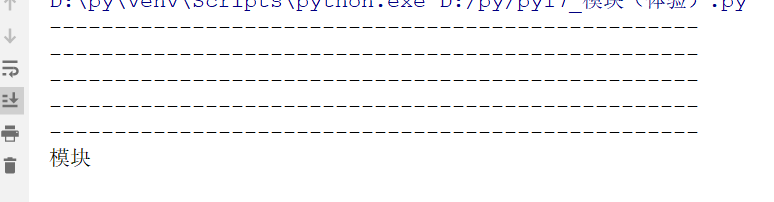19，列表

```````# List 列表 是python中使用最频繁的数据类型，在其他语言中通常叫做 数组
# 专门用来储存一串信息，用[]定义，数据 之间用 ，
# 列表的 索引 从0开始，（数据在列表的位置编号，也被称 下标）`
name_list = ["a","b","c"]
# 取值和取索引
print(name_list)
print(name_list.index("b"))  # index“索引”
# 修改
name_list = "d"
# 增加
name_list.append("e")
#    append 可以向列表末尾追加数据
name_list.insert(3,"f")
#    insert 可以在列表指定索引位置插入
temp_list = ["t","y","u","a","l"]
name_list.extend(temp_list)
#    extend 可以将其他列表内容，追加到当前列表的末尾
# 删除
name_list.remove("a")
#    remove 可以从列表中删除指定的数据，且是第一次出现的数据
name_list.pop()
#    pop 默认情况下可以将列表最后一个元素删除
name_list.pop(0)
#    pop 也可以将列表中指定 数据的索引 删除
del name_list 
#    del 关键字本质上是用来将一个变量从内存中删除
# name_list.clear()
#    clear 可以清空列表
# 统计
list_let = len(name_list)
print("列表中包含 %d 个元素" % list_let)
#    len 函数可以统计列表元素的总数
count = name_list.count("y")
print("y出现了 %d 次" % count)
#    count 可以统计列表某一个数据出现的次数
print(name_list)
``````
``````a
1

y出现了 1 次
['d', 'f', 't', 'y', 'u', 'a']
``````
``````name_list = ["rr","bb","uu","dd","ee"]
num_list = [5,6,7,2,8]
# 升序
#name_list.sort()
#num_list.sort()

# 降序
#name_list.sort(reverse=True)
#num_list.sort(reverse=True)

# 逆序（反转）
#name_list.reverse()
#num_list.reverse()

print(name_list)
print(num_list)
``````
``````['rr', 'bb', 'uu', 'dd', 'ee']
[5, 6, 7, 2, 8]
``````

20，for（迭代遍历）

```````# 遍历 就是 从头到尾 依次 从列表中获取数据，`
`#   在循环体内部 针对 每一个元素。执行相同的操作`
`# python为了提高列表遍历效率，专门提供迭代iteration遍历`
name_list = ["张三","李四","王五","赵六"]
"""

my_name 变量中，在循环体内部可以访问到当前这一次获取的数据

for my_name in 列表变量:
print("我的名字叫 %s" % my_name)

"""
for my_name in name_list:
print("我的名字叫 %s" % my_name)
``````
``````我的名字叫 张三

``````

21，元组

```````# Tuple 元组 以列表类似，不同之处在于元组的元素不能修改
#   元组表示多个元素组成的序列
#  用于存储一串信息，数据之间用，分隔  用（）定义`
info_tuple = ("zhangsan",28,28,2.33)

# 取值索引
print(info_tuple)
print(info_tuple.index(2.33))

# 统计计数
print(info_tuple.count("zhangsan"))
#　统计元组中包含元素的个数
print(len(info_tuple))
``````
``````zhangsan
3
1
4
``````

```````# 函数的参数和返回值，一个函数可以接收任意多个参数，或者一次返回多个数据
# 格式字符串，格式化字符串后边的 （） 本质上就是一个元组
# 让列表不可以被修改 ，以保存数据安全`
info_tuple = ("小明",18,177)
print("%s 年龄是 %d 身高是 %.2f" %info_tuple)
info_str = "%s 年龄是 %d 身高是 %.2f" %info_tuple
print(info_str)
`# 元组和列表之间的转换
# 使用list函数 可以把元组转换成列表
# 使用 tuple 函数可以把列表转换成元组`
``````

```````# 取值 就是从元组中获取存储在指定位置的数据
# 遍历 就是从头到尾 依次从元组中获取数据`
info_tuple = ("zhangsan",30,4.44)

# 使用迭代遍历元组
for my_info in info_tuple:

# 使用格式字符串拼接 my_info 这个变量不方便！
# 因为元组中通常保存的数据类型是不同的
print(my_info)
`# 在Python中，可以使用 for 循环遍历所有非数字型类型的变量：列表，元组，字典，字符串
# 在实际开发中，除非 能够确认元组中的数据类型，否则针对元组的循环遍历需求并不是很多`
``````
``````zhangsan
30
4.44
``````

22，字典

dictionary和列表的区别

``````xiaoming = {
"name": "小明",
"age":18,
"gender":True,
"height":175,
"weight":75}
print(xiaoming)
`# 字典是一个无序的数据集合，使用print输出字典时，
# 输出顺序和定义的顺序不一致`
``````
``````{
'name': '小明', 'age': 18, 'gender': True, 'height': 175, 'weight': 75}
``````
``````xiaoming_dict = {
"name":"小明"}

# 取值
print(xiaoming_dict["name"])
# 增加/修改
xiaoming_dict["age"]=18
xiaoming_dict["name"]="xixixxi"

# 删除
xiaoming_dict.pop("name")

print(xiaoming_dict)
``````
``````小明
{
'age': 18}
``````
``````xiaoming_dict = {
"name":"xiaoming",
"age":18}
# 统计键值对数量
print(len(xiaoming_dict))
# 合并字典
temp_dict = {
"height":176,
"age":25}

xiaoming_dict.update(temp_dict)
`# 如果被合并的字典中包含已经存在的键值对，会覆盖原有键值对`
# 清空字典
xiaoming_dict.clear()

print(xiaoming_dict)
``````
``````2
{
}
``````

``````xiaoming_dict = {
"name":"xiaoming",
"qq":"1234123",
"phone":"12342"}
# 迭代遍历
# 变量 k 是每一次循环中，获取到的键值对的 key
for k in xiaoming_dict:
print("%s - %s"%(k,xiaoming_dict[k]))
``````
``````name - xiaoming
qq - 1234123
phone - 12342
``````

```````# 使用多个键值对，存储描述一个 物体 的相关信息--描述更复杂的数据信息
# 将多个字典 放在一个列表中，在进行遍历，在循环体内部对每一个字典进行 相同的处理`
card_list = [
{
"name":"zhangsan",
"age":19,
"qq":"12314",
"phone":"123123"},
{
"name":"asdf",
"age":123,
"qq":"23123",
"phone":"1232312"},
{
"name":"dasda"},
{
"name":"asdfasd"},
]

for card_info in card_list:
print(card_info)
``````
``````{
'name': 'zhangsan', 'age': 19, 'qq': '12314', 'phone': '123123'}
{
'name': 'asdf', 'age': 123, 'qq': '23123', 'phone': '1232312'}
{
'name': 'dasda'}
{
'name': 'asdfasd'}
``````

23，字符串

```````# 字符串就是一串字符，是编程语言中表示文本的数据类型
# 在Python 中可以使用 一对双引导 " 或者一对单引号 ' 定义一个字符串
# 大多数情况下使用 " 来定义字符串`
str1 ='我的外号是"asdf"'
print(str1)
`# 虽然可以使用 \" 或者 \' 做字符串转义，但是在实际开发中
# 如果字符串内部需要 " ,可以使用 ' 定义字符串
# 反之也可以
#　可以使用　索引　获取字符串中指定位置的字符，索引计数从０开始`
str2 = "hello python"
print(str2)
`# 也可以使用 for 循环遍历字符串中每一个字符`
for char in str1:
print(char)
``````
``````我的外号是"asdf"
p

"
a
s
d
f
"
``````
``````hello_str = "hello hello"
# 统计字符串长度
print(len(hello_str))
# 统计某一子字符串出现的次数
print(hello_str.count("h"))

# 某一子字符串出现的位置
print(hello_str.index("h"))
``````
``````11
2
0
``````

`isspace()`判断空白字符如果只包含空格，则返回 True（转义字符为空白字符）
`isalnum()`判断至少有一个字符并且所有字符都是字母或数字，则返回 True
`isalpha()`判断至少有一个字符并且所有字符都是字母，则返回 True

``````space_str = "     \t\n\r"
print(space_str.isspace())
``````
``````True
``````

`isdecimal()`判断只包含数字，则返回 True，全角数学
`isdigit()`判断只包含数字，则返回 True，全角数学，（1），\u00b2（平方）
`isnumeric()`判断只包含数字，则返回 True，全角数学，中文数字
`都不能判断小数`

``````num_str = "1"
print(num_str.isdecimal())
print(num_str.isdigit())
print(num_str.isnumeric())
``````
``````True
True
True
``````

`istitle()`判断是标题化的（每个首字母大写），则返回 True
`islower()`判断包含至少一个区分大小写的字符，并且所有这些（区分大小写）的字符都是小写，则返回 True
`isupper()`判断包含至少一个区分大小写的字符，并且所有这些（区分大小写）的字符都是大写，则返回 True

``````hello_str = "hello world"
`# startswith()判断是否已指定字符串开始`
print(hello_str.startswith("he"))
`# endswith()判断是否已指定字符串结束`
print(hello_str.endswith("ld"))
`# find()查找指定字符串，如果指定的字符串不存在，会返回-1`
print(hello_str.find("l"))
`# rfind()同find，但是是从右侧开始
# index()同find，但是如果指定字符串不存在，会报错
# rindex()同index，但是是从右侧开始
# replace()替换字符串,执行完成之后，会返回一个新的字符串`
print(hello_str.replace("world","asdfa"))
`# 且不会修改原有字符串的内容`
print(hello_str)
``````

`capitalize()`把字符串第一个字符大写
`title()`把字符串每个单词首字母大写
`lower()`将字符串中所有大写字符转换为小写
`upper()`将字符串中的小写字母为大写
`swapcase()`翻转字符串中的大小写
`ljust()`左对齐
`rjust()`右对齐
`center()`居中对齐
`lstrip()`截掉字符串左边开始的空白字符
`rstrip()`截掉字符串右边末尾的空白字符
`strip()`截掉字符串左右两边的字符

``````poem = ["\t\n登鹳雀楼",
"王之涣",
"白日依山尽\t\n",
"黄河入海流",
"欲穷千里目",
"更上一层楼"]
for poem_str in poem:
# 先使用strip去除空白字符，在使用center居中
print("|%s|" % poem_str.strip().center(10,"　"))
``````
``````|　　　登鹳雀楼　　　|
|　　　王之涣　　　　|
|　　白日依山尽　　　|
|　　黄河入海流　　　|
|　　欲穷千里目　　　|
|　　更上一层楼　　　|
``````

### 【教程】用安卓厨房制作你自己的卡刷包！你也可以是Romer ！_android kitchen-程序员宅基地

ROM定制工具——dsixda’s Android Kitchen是由xda-developers论坛的一位来自加拿大的大神做出来的。这是一个定制rom的神器！！ 在这里向作者表示崇高的敬意——原帖地址：http://forum.xda-developers.com/showthread.php?t=633246 厨房下载地址： https://github.com/dsixda/Andro_android kitchen

### ibatis扩展支持主键生成的方法（非数据库方式）-程序员宅基地

﻿﻿问题： 持久层使用ibatis保存数据进数据库的时候，会用到一些序列（sequence）的数据通常被用于数据表主键唯一标识。Ibatis框架中主要通过selectKey节点完成sequence值生成和返回，但selectKey仅局限于数据库sql语句使用，不适用于想通过某个类方法自定义生成序列（主键）的方式。这里我们在了解selectKey运行流程的基础上